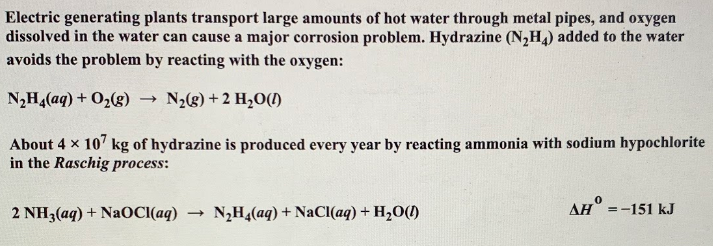# Electric generating plants transport large amounts of water through metal pipes, and oxygen dissolved in the water can cause a major corrosion problem. Hydrazine (N2H4) added to the water avoids the problem by reacting with the oxygen: N2H4(aq) + O2(g) → N2(g) + 2 H2O(l) About 4x10^7 kg of hydrazine is produced every year by reacting ammonia with sodium hypochlorite in the Raschig process: 2 NH3(aq) + NaOCl(aq) → N2H4(aq) + H2O(l) Δh degrees =-151 kJ b) What is the heat released when aqueous N2H4 is added to 5.25x10^3 L of plant water that is 2.50X10^-4 M O2? Enter your answer in scientific notation. Δh N2H4 = 35.5 kJ/mol